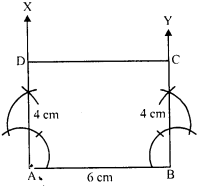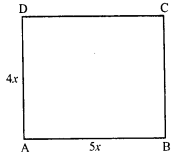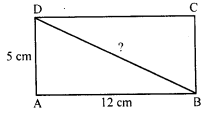×#### Thank you for registering.

One of our academic counsellors will contact you within 1 working day.

Click to Chat

1800-1023-196

+91-120-4616500

CART 0

• 0

MY CART (5)

Use Coupon: CART20 and get 20% off on all online Study Material

ITEM
DETAILS
MRP
DISCOUNT
FINAL PRICE
Total Price: Rs.

There are no items in this cart.
Continue Shopping• Complete JEE Main/Advanced Course and Test Series
• OFFERED PRICE: Rs. 15,900
• View Details

```Chapter 17: Understanding Shapes III Special Types of Quadrilaterals Exercised – 17.3

Question: 1

Which of the following statements are true for a rectangle?

(i) It has two pairs of equal sides.

(ii) It has all its sides of equal length.

(iii) Its diagonals are equal.

(iv) Its diagonals bisect each other.

(v) Its diagonals are perpendicular.

(vi) Its diagonals are perpendicular and bisect each other.

(vii) Its diagonals are equal and bisect each other.

(viii) Its diagonals are equal and perpendicular, and bisect each other.

(ix) All rectangles are squares.

(x) All rhombuses are parallelograms.

(xi) All squares are rhombuses and also rectangles.

(xii) All squares are not parallelograms.

Solution:

(i) True.

(ii) False. (Only pair of opposite sides is equal)

(iii) True

(iv) True

(v) False (Diagonals are not perpendicular)

(vi) False (Diagonals are not perpendicular to each other)

(vii) True

(viii) False (Diagonals are equal but not perpendicular)

(ix) False (All rectangles are not square but a special type can be a square)

(x) True

(xi) True

(xii) False (All squares are parallelograms because their opposite sides are parallel and equal)

Question: 2

Which of the following statements are true for a square?

(i) It is a rectangle.

(ii) It has all its sides of equal length.

(iii) Its diagonals bisect each other at right angle.

(iv) Its diagonals are equal to its sides.

Solution:

(i) True

(ii) True

(iii) True

(iv) False (Each diagonal of a square is greater than its side)

Question: 3

Fill in the blanks in each of the following so as to make the statement true:

(i) A rectangle is a parallelogram in which

(ii) A square is a rhombus in which

(iii) A square is a rectangle in which

Solution:

(i) A rectangle is a parallelogram in which one angle is right angle.

(ii) A square is a rhombus in which one angle is right angle.

(iii) A square is a rectangle in which adjacent sides are equal.

Question: 4

A window frame has one diagonal longer than the other. Is the window frame a rectangle? Why or why not?

Solution:

No, it is not a rectangle as rectangle has diagonals of equal length.

Question: 5

In a rectangle ABCD, prove that AACB = ACAD.

Solution:In rectangle ABCD, AC is its diagonal.

AB = CD (Opposite sides of a rectangle)

AC = AC (Common)

Question: 6

The sides of a rectangle are in the ratio 2: 3 and its perimeter is 20 cm. Draw the rectangle.

Solution:

Perimeter of a rectangle = 20 cm

Ratio in the sides = 2: 3

Then length (b) = 3x

Perimeter = 2 (I + b)20 = 2 (2x + 3x)

4x + 6x = 20

10x = 20

x = 20/10 = 2

Length = 3x = 3 x 2 = 6

and breadth = 2x = 2 x 2 = 4 cm

Steps of construction:

(i) Draw a line segment AB = 6 cm.

(ii) At A and B draw perpendicular AX and BY

(iii) Cut off from AX and BY, AD = BC = 4 cm.

(iv) Join CD.

Then ABCD is the required rectangle.

Question: 7

The sides of a rectangle are the ratio 4: 5. Find its sides if the perimeter is 90 cm.

Solution:

Perimeter of a rectangle = 90 cm.Ratio in sides = 4: 5

Let first side = 4x

Then second side = 5x

Perimeter = 2(1 + b)

2 (4x + 5x) = 90

2 x 9x = 90

18x = 90

x = 5

First side = 4x = 4 x 5 = 20 cm

and second side = 5x = 5 x 5 = 25 cm

Question: 8

Find the length of the diagonal of a rectangle whose sides are 12 cm and 5 cm.

Solution:

In rectangle ABCD, AB = 12 cm and AD = 5 cmBD is its diagonal.

Now, in right angled ∆ABD,

BD2 = AB2 + AD2 (Pythagoras theorem)

= (12)2 + (5)2 = 144 + 25 = 169 = (13)2

BD = 13 cm

Length of diagonal = 13 cm
```### Course Features

• 728 Video Lectures
• Revision Notes
• Previous Year Papers
• Mind Map
• Study Planner
• NCERT Solutions
• Discussion Forum
• Test paper with Video Solution